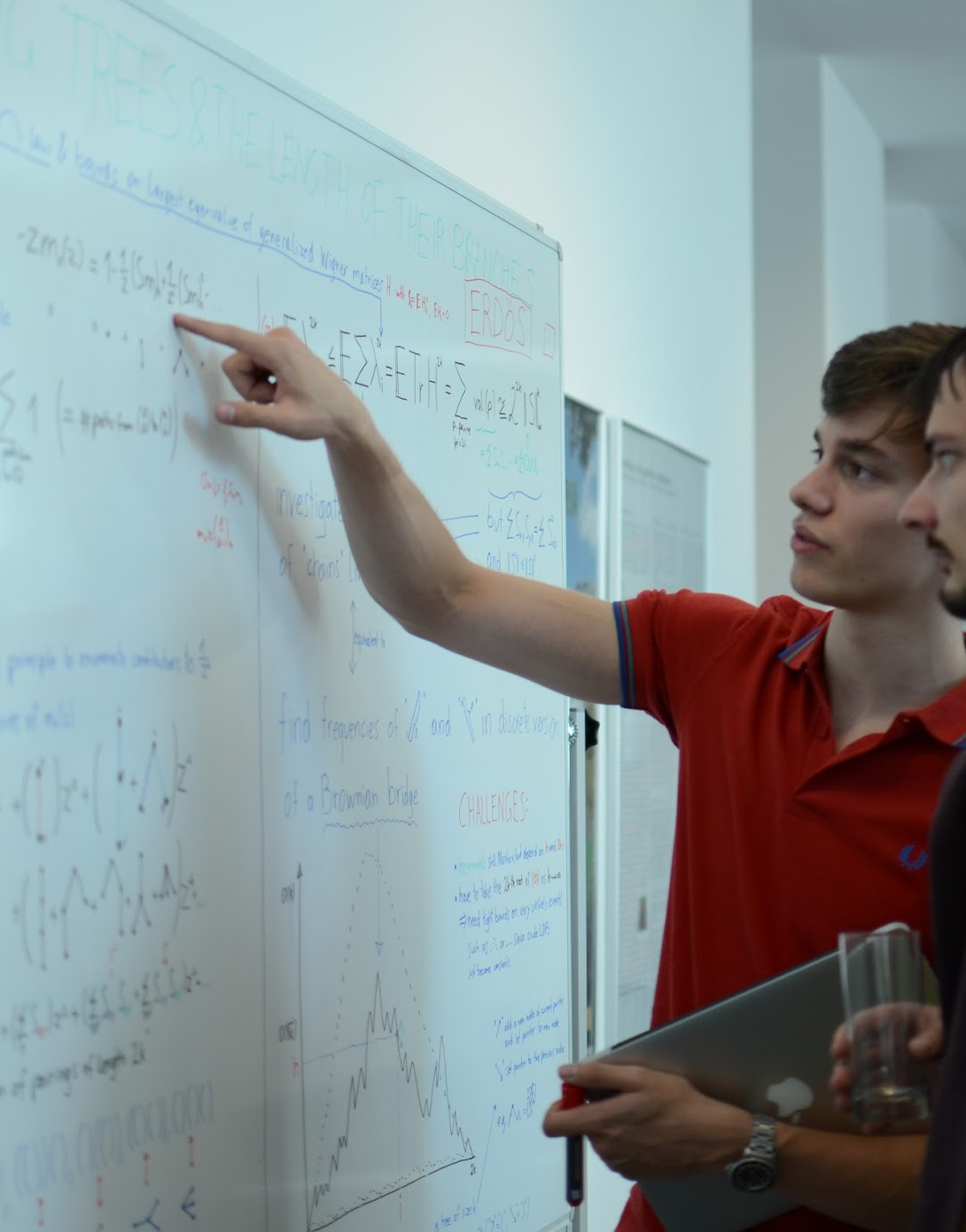# Peter Mühlbacher

I am a fourth year PhD student under supervision of Daniel Ueltschi.

Email: Peter "dot" Muhlbacher "at" warwick "dot" ac "dot" uk

Website: peter.muehlbacher.me

## Research Interests

My main interests are quantum spin systems and in particular their graphical representations. One toy example is the random interchange model: Start with a graph and put a label on each vertex. At every time step one edge is chosen at random and the adjacent labels are swapped. This defines a random permutation. Various quantities of interest (to physicists) turn out to be related to the permutation cycle structure of this permutation. Questions I am interested in include

• Is there a macroscopic permutation cycle at a given time?
• If there is a macroscopic permutation cycle at time t, could it be that there are only microscopic cycles at time s (s>t)?
• What does the cycle structure look like?

They depend strongly on the geometry of the underlying graph.

One might see this as an SN-valued Markov process. The field of mixing times addresses the question how long it takes until one approaches the equilibrium distribution (typically in total variation distance) and whether there is a sharp transition. It turns out, however, that if one only looks at the cycle structure, one converges to the equilibrium, the Poisson-Dirichlet distribution, on much shorter time scales.

Some questions can be resolved by considering a natural coupling to a percolation process. Going away from the toy model to the models that are actually of interest to physicists bears a striking resemblance to going from classical percolation to the random cluster model.

Alternatively, the time evolution can be seen as a simple random walk on the Cayley graph of the symmetric group with generators being transpositions supported on the edges of the graph. In this case representation theory (essentially Fourier analysis on non-commutative spaces) provides some insights.

Yet another way to understand the random interchange model is by considering a certain non-Markovian random walk and determining whether it is transient/recurrent. To this end I am interested in techniques devised to prove transience for simple random walks, like electrical networks.

If you have ideas/advice/questions on anything above in bold (or anything else), I would love to hear about it!